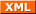# Christoph's last Weblog entries

### Entries from April 2019.

7th April 2019

This is FAUST playing CTF again, this time midnightsun.

Team: FAUST
Crew: siccegge

OK so we're looking at the EZDSA service. This is a signature service and the task is essentially to recover the signing key. Code is reproduced below.

#!/usr/bin/python2
from hashlib import sha1
from Crypto import Random
from flag import FLAG

class PrivateSigningKey:

def __init__(self):
self.gen = 0x44120dc98545c6d3d81bfc7898983e7b7f6ac8e08d3943af0be7f5d52264abb3775a905e003151ed0631376165b65c8ef72d0b6880da7e4b5e7b833377bb50fde65846426a5bfdc182673b6b2504ebfe0d6bca36338b3a3be334689c1afb17869baeb2b0380351b61555df31f0cda3445bba4023be72a494588d640a9da7bd16L
self.key = int(FLAG.encode("hex"), 16)

def sign(self, m):

def bytes_to_long(b):
return long(b.encode("hex"), 16)

h = bytes_to_long(sha1(m).digest())
assert(bytes_to_long(m) % (self.q - 1) != 0)

k = pow(self.gen, u * bytes_to_long(m), self.q)
r = pow(self.gen, k, self.p) % self.q
s = pow(k, self.q - 2, self.q) * (h + self.key * r) % self.q
assert(s != 0)

return r, s


The outer service was not provided but you could pass in base64 encoded byte arrays and got back r and s as already indicated. Looking at the final computation for s we notice that given $$(h + k * r)$$ and $$h, r$$ we can easily recover $$k$$. For this to work it would be convenient if the first term ends up being 1. Unfortunately, the easiest way to get there is prevented: $$g^{q-1} = 1$$. Fortunately this is not the only exponent where this works and a good candidate is $$(q-1 / 2)$$.

pow(gen, (q-1)//2, q)
1


From there the only thing left is solving $$s = (h + k * r)$$. Fortunately gmpy has the solution prepackaged again: divm. So we proceed by getting a valid "signature" on $$(q-1 / 2)$$. The rest is simple calculation:

#!/usr/bin/python3
sha1(binascii.unhexlify("%x" % ((q-1)//2))).hexdigest()
'e6d805a06977596563941c1e732e192045aa49f0'

base64.b64encode(binascii.unhexlify("%x" % ((q-1)//2)))

gmpy2.divm(s-h, r, q)
mpz(39611266634150218411162254052999901308991)

binascii.unhexlify("%x" % 39611266634150218411162254052999901308991)
b'th4t_w4s_e4sy_eh?'


OK so why does $$(q-1 / 2)$$ work? Essentially, the field defined $$F_q$$ -- calculations mod q -- has q elements additively and $$q-1$$ elements multiplicatively(and we're considering exponentiation as repeated multiplication). Therefore it contains cyclic subgroups for all factors of $$q-1$$ and for every element $$e$$, $$e^o = 1$$ where o is the order of the subgroup that element belongs to. as the generator is trivially not $$-1$$ -- the subgroup of size 2 -- $$(q-1 / 2)$$ must be a multiple of the generated group's order.

Tags: crypto, ctf, faust, python, security, writeup.Created by Chronicle v4.6